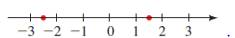# the distance between the two numbers on the given number line.### Precalculus: Mathematics for Calcu...

6th Edition
Stewart + 5 others
Publisher: Cengage Learning
ISBN: 9780840068071### Precalculus: Mathematics for Calcu...

6th Edition
Stewart + 5 others
Publisher: Cengage Learning
ISBN: 9780840068071

#### Solutions

Chapter 1.1, Problem 72E
To determine

## To find: the distance between the two numbers on the given number line.

Expert Solution

The distance between the two numbers is 4 .

### Explanation of Solution

Given information:

A number line is given asFormula used:

Distance between two numbers a and b is given by

d(a,b)=|ab|=|ba|=d(b,a)

Absolute value property:

For any real number a,

|a|=a0|a|=|a|=a

Calculation:

Consider the given number line.Two numbers on the number line is 2.5 and 1.5 .

Distance between the numbers can be calculated as shown below:

d(a,b)=|ab|=|2.51.5|=|4|

Now, compare the distance with |a| :

|a|=|4|a=4

Now, by absolute value property:

|a|=a0

Therefore, |4|=4

Hence, distance between the numbers is 4 units.

### Have a homework question?

Subscribe to bartleby learn! Ask subject matter experts 30 homework questions each month. Plus, you’ll have access to millions of step-by-step textbook answers!